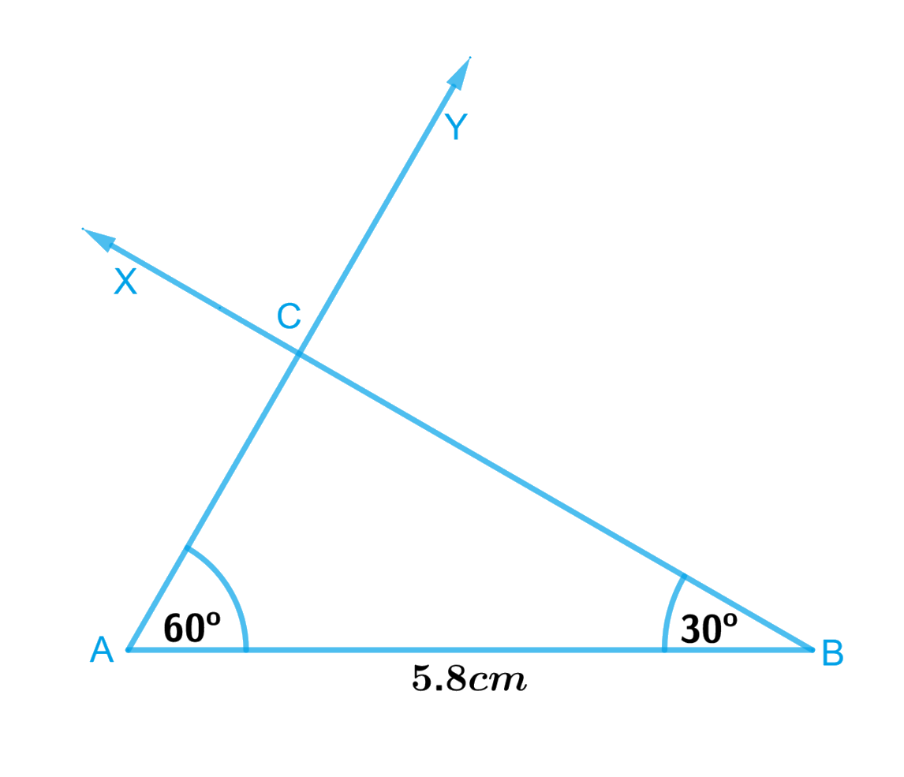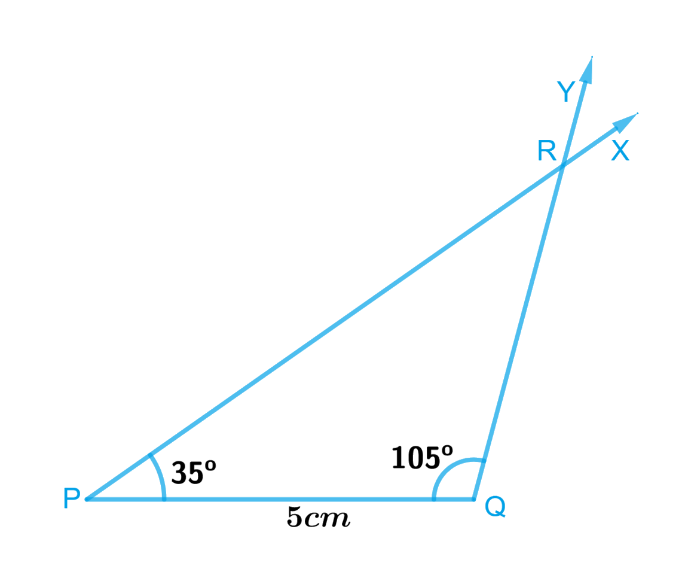# Exercise 10.4 Practical-Geometry -NCERT Solutions Class 7

Go back to  'Practical Geometry'

## Chapter 10 Ex.10.4 Question 1

Construct $$ΔABC$$, given $$m∠A = 60^\circ$$, $$m∠B = 30^\circ$$ and $$AB = 5.8\,\rm{ cm}$$.

### Solution

What is known?

Length of side of a $$ΔABC$$, $$AB = 5.8\,\rm{ cm}$$  and  $$∠A = 60^\circ$$, $$∠B = 30^\circ$$.

To construct:

A triangle $$ΔABC$$, given $$∠A = 60^\circ$$, $$∠B = 30^\circ$$ and $$AB = 5.8\,\rm{ cm}$$.

Reasoning:

To Construct a $$ΔABC$$ such that $$∠A = 60^\circ, ∠B = 30^\circ$$ and $$AB = 5.8 \,\rm{cm}$$., follow the steps given below.

Steps:Steps of construction:

1. Draw a line segment $$AB$$ of length $$5.8\,\rm{ cm}.$$
2. At $$A$$, draw ray $$AY$$ making $$60^\circ$$ with $$AB$$.
3. At $$B$$, draw ray $$BX$$ making $$30^\circ$$ with $$AB$$.
4. Rays $$BX$$ and $$AY$$ will intersect at point $$C$$.

Triangle $$ABC$$ is now completed.

## Chapter 10 Ex.10.4 Question 2

Construct $$ΔPQR$$  if  $$PQ = 5 \rm{cm}$$, $$m∠PQR = 105^\circ$$ and $$m∠QRP = 40^\circ$$. (Hint: Recall angle-sum property of a triangle).

### Solution

What is known?

In triangle $$PQR$$ , $$PQ = 5 \rm{cm}$$, $$∠PQR = 105^\circ$$ and $$∠QRP = 40^\circ$$.

To construct:

A triangle $$ΔPQR$$ if $$PQ = 5 \rm{cm}$$, $$∠PQR = 105^\circ$$ and $$∠QRP = 40^\circ$$.

Reasoning:

We will use angle-sum property of a triangle to find the measure of $$∠RPQ$$
By angle sum property of a triangle,
$$∠PQR + ∠QRP + ∠RPQ = 180^\circ.$$
$$105^\circ + 40^\circ + ∠RPQ = 180^\circ.$$
So, $$∠RPQ = 35^\circ$$
Now, let’s Construct $$ΔPQR$$ such that $$PQ = 5\, {\rm{cm}} , ∠PQR = 105^\circ$$ and $$∠RPQ = 35^\circ$$, with the steps given below

Steps:Steps of construction

1. Draw a line segment $$PQ$$ of length $$5 \, \rm{cm}.$$
2. At $$P$$, draw $$PX$$ making $$35^\circ$$ with $$PQ.$$
3. At $$Q,$$ draw $$QY$$ making an angle of $$105^\circ$$ with $$PQ.$$
4. $$PX$$ and $$QY$$ will intersect at point $$R.$$

$$PQR$$ is the required triangle.

## Chapter 10 Ex.10.4 Question 3

Examine whether you can construct $$ΔDEF$$ such that $$EF = 7.2\,\rm{ cm},$$ $$m∠E = 110^\circ$$ and $$m∠F = 80^\circ.$$Justify your answer.

### Solution

What is known?

Length of one side of a triangle and measure of two angles.

What is unknown?

Whether a triangle be constructed with the given values of length and angles.

Reasoning:

By using the angle sum property, we can find out the third angle. If the angle sum property is followed, then it is possible to construct a triangle and if not then we cannot construct a triangle.

Steps:

We will use angle-sum property of a triangle to find the measure of $$∠D$$

By angle sum property,

$$∠E +∠F + ∠D =$$ $$180^\circ .$$

$$110^\circ + 80^\circ + ∠D =$$ $$180^\circ.$$

$$190^\circ + ∠D$$ $$=$$ $$180^\circ .$$

So, $$∠D = -10^\circ$$

Angle of $$-10^\circ$$ is not possible, thus we cannot construct triangle $$ΔDEF.$$

Related Sections
Related Sections
Instant doubt clearing with Cuemath Advanced Math Program